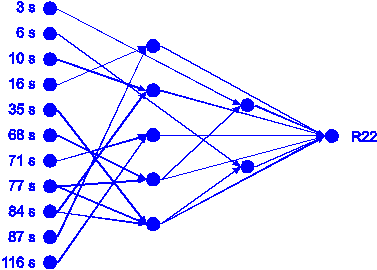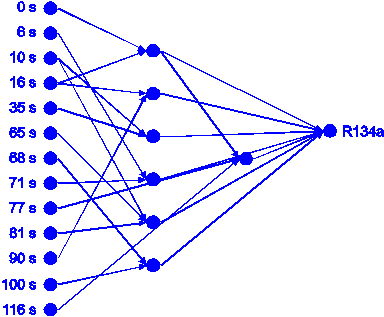Home News About Me Ph. D. Thesis Abstract Table of Contents 1. Introduction 2. Theory  Fundamentals of the Multivariate Data Analysis 3. Theory  Quantification of the Refrigerants R22 and R134a: Part I 4. Experiments, Setups and Data Sets 5. Results  Kinetic Measurements 6. Results  Multivariate Calibrations 7. Results  Genetic Algorithm Framework 8. Results  Growing Neural Network Framework 8.1. Modifications of the Growing Neural Network Algorithm 8.2. Application of the Growing Neural Networks 8.3. Growing Neural Network Algorithm Frameworks 8.4. Applications of the Growing Neural Network Frameworks 8.5. Conclusions and Comparison of the Different Methods 9. Results  All Data Sets 10. Results  Various Aspects of the Frameworks and Measurements 11. Summary and Outlook 12. References 13. Acknowledgements Publications Research Tutorials Downloads and Links Contact Search Site Map Print this Page## 8.2.   Application of the Growing Neural Networks

For the application of the growing neural net algorithm, the calibration data set was split into a training (80 %) and a monitor (20%) subset by a random subsampling procedure (see section 2.4). Using the stopping criterion of 0.1% minimal error decrease the growing network algorithm built the network for R22 shown in figure 50 with 11 input neurons, 22 links and 7 hidden neurons organized in 2 hidden layers. For R134a the network consisted of 13 input neurons, 23 links and 7 hidden neurons organized in 2 hidden layers shown in figure 51.figure 50:  Neural network built by the first run of the growing neural network algorithm for R22.figure 51:  Neural network built by the first run of the growing neural network algorithm for R134a.

These network topologies were trained using the complete calibration data set and then predicted the concentrations of the external validation data. According to table 4 in section 8.5, the grown neural networks predicted the external validation data not used for the network growing process significantly better than non-optimized static neural networks and no significant gap between the predictions of the calibration and validation data is visible.

Yet, similar to the application of single run genetic algorithms for the optimization of neural networks (see section 2.8.9), the topology of the grown networks depends highly on the partitioning of the data set. A second run of the algorithm with differently subsampled training and monitor data subsets resulted in other network topologies for both analytes. The network for R 22 of this second run is shown in figure 52. Although several substructures, which are printed in green, are equal to the network shown in figure 50, both networks also show significant differences, which are printed in blue in figure 52. In principle, these differences of the network topology are not necessarily bad as for a given set of input variables the approximation of a functional relationship between the input and the response variables can be performed by a neural network on nearly uncountable ways. Yet, the selection of different variables during different runs is by far more problematic. For example, the second network uses the time points 13 s, 22 s and 29 s instead of 16 s and 116 s as input variables, which are printed in red in figure 52. The selection of different variables irreversibly changes the possibilities of the functional mapping and significantly influences the quality of calibration. As can be seen in table 4, the predictions of the validation and calibration data differ for the nets built during the different runs whereby the growing neural nets performed generally better for the validation data than the static neural nets during several runs. Also, the network of the second run for R134a with 10 input neurons, 18 links and 5 hidden neurons organized in 1 hidden layer differs significantly from the network of the first run for R134a in respect to the topology and even worse in respect to the selected variables.

Similar to the single runs of genetic algorithms the topology and more important the selection of the variables are representative for only one partitioning of the data set into calibration and monitor data set and not for the complete data set. Analogous to the framework of the genetic algorithms (section 7.2), two frameworks are proposed in the next section to make the variable selection of the growing neural networks less sensitive to the partitioning of the data into different subsets and to different random initial weights. In section 8.4, these two frameworks are applied to the refrigerant data sets resulting in improved calibrations.figure 52:  Neural network built by the second run of the growing neural network algorithm for R22. Elements equal to the network of the first run are printed in green.

 Page 108 © Frank Dieterle, 03.03.2019